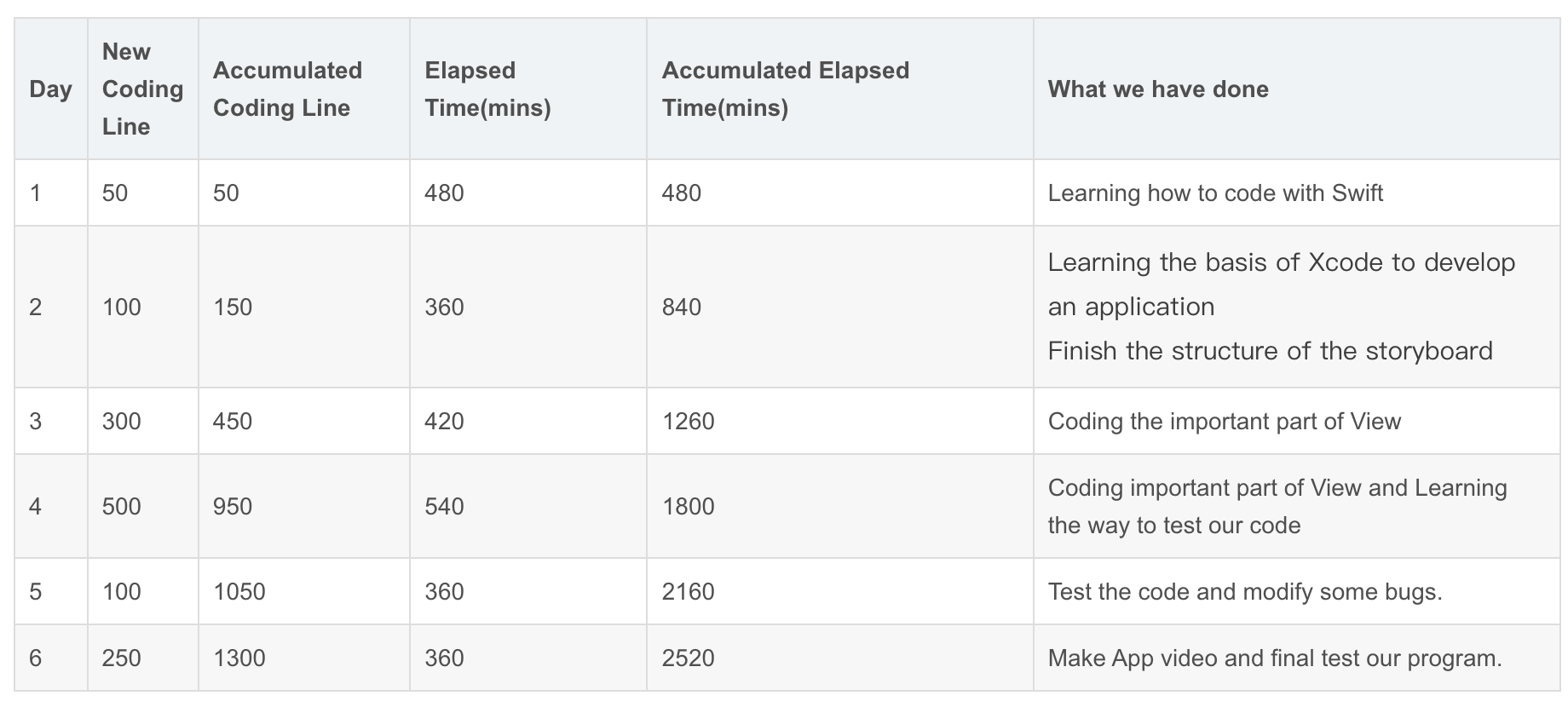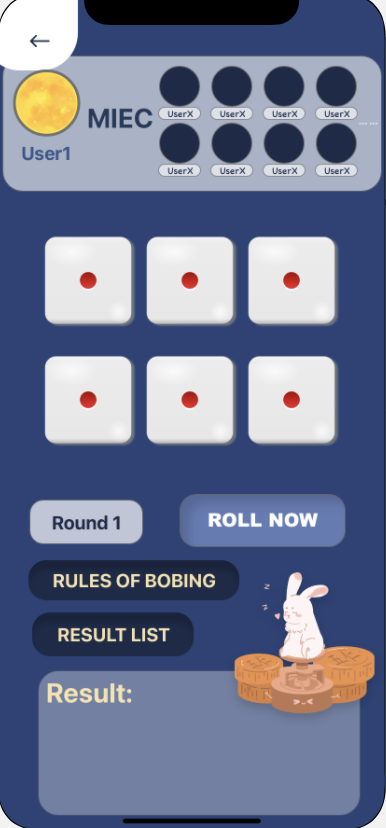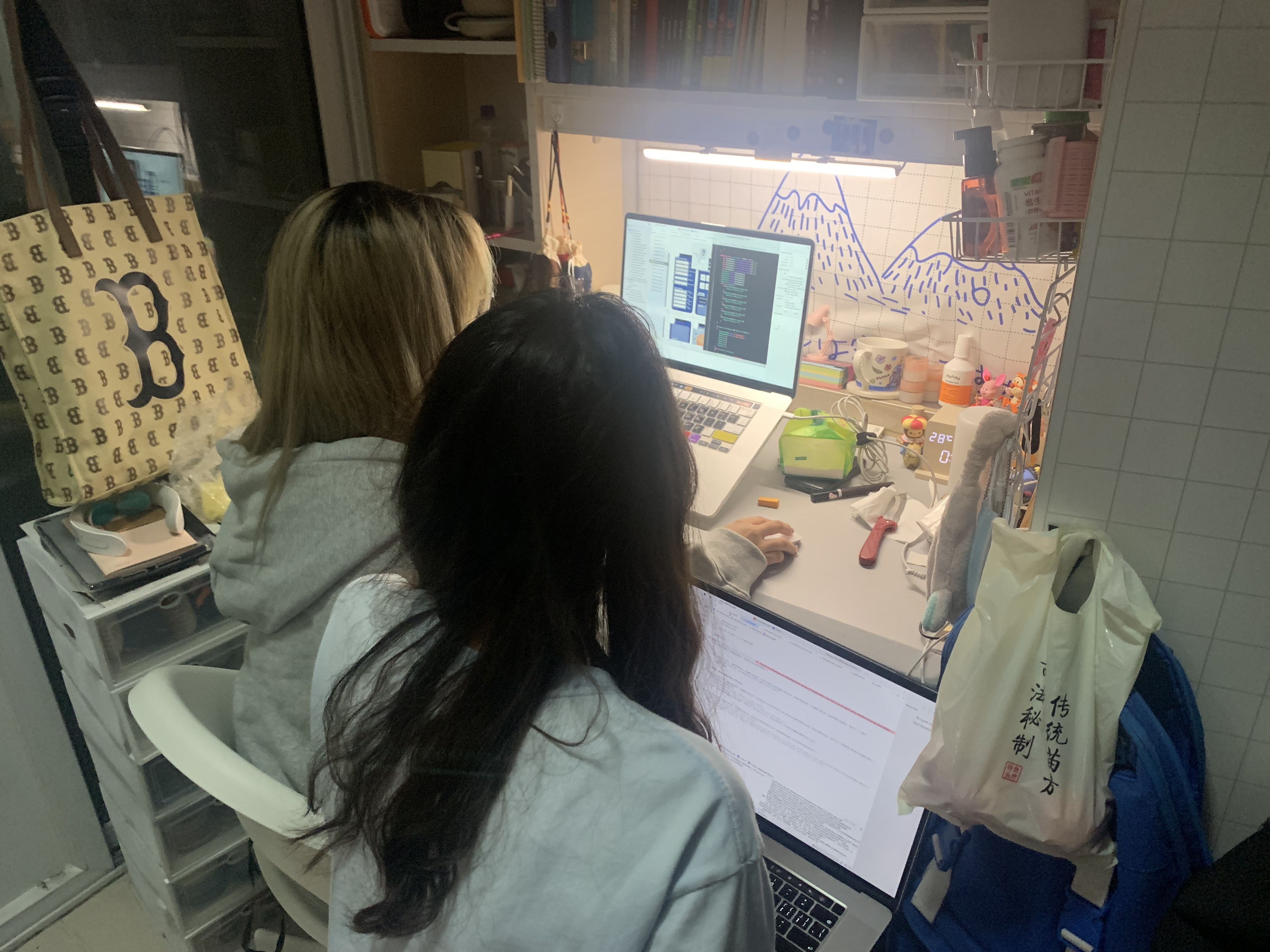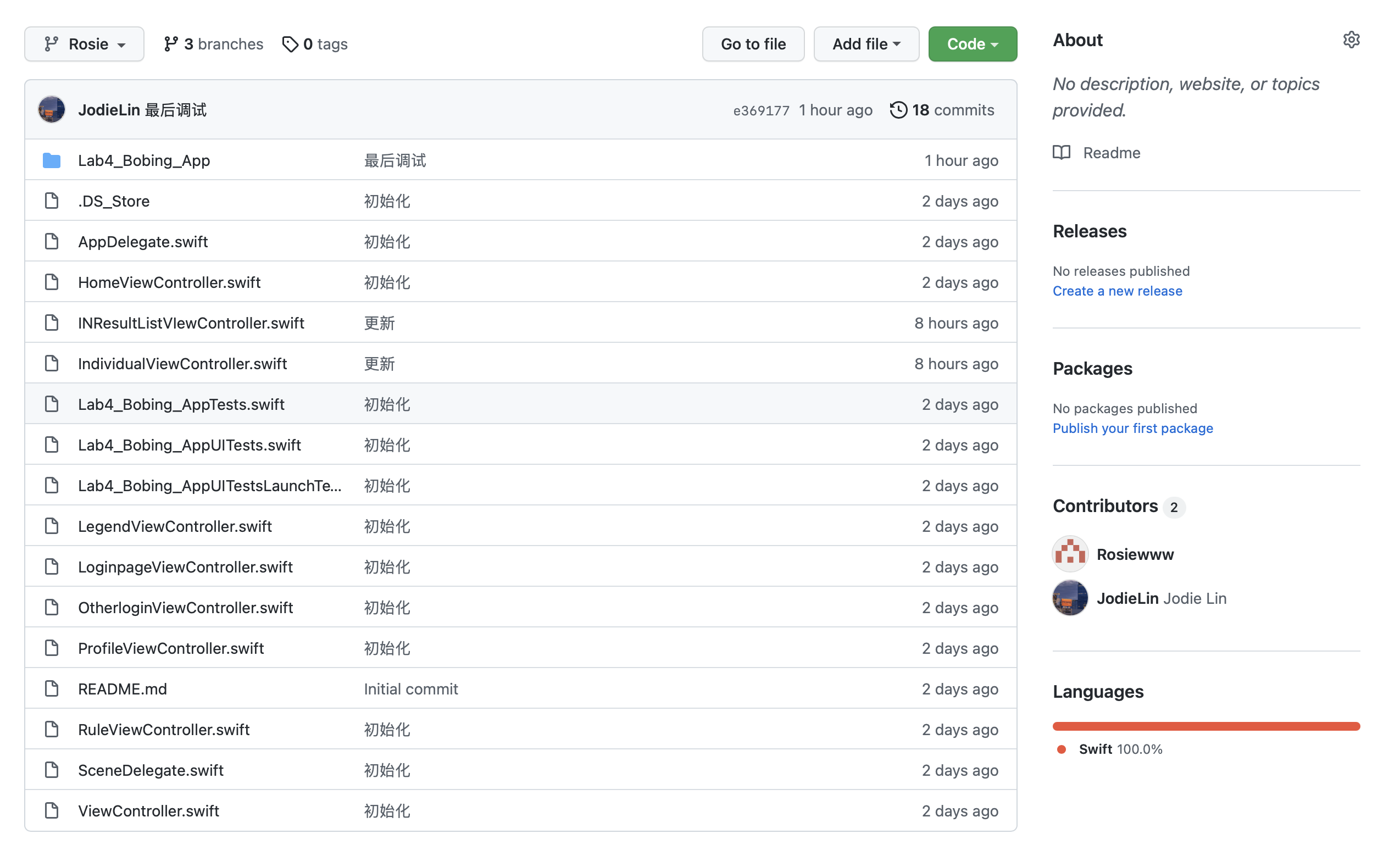# EE308_Lab4

 The Link Your Class https://bbs.csdn.net/forums/MUEE308FZ?category=0 The Link of Requirement of This Assignment https://bbs.csdn.net/topics/601188617 MU STU ID and FZU STU ID 19105819_831901130 Teammate's MU STU ID and FZU STU ID 19105754_831901114 Teammate's blog link https://blog.csdn.net/jodie__lin?type=bbs GitHub link https://github.com/Jodie-Rosie/Lab4_MIEC_Bobing Video demo link https://www.bilibili.com/video/BV1344y1x7CC?share_source=copy_web

1. PSP form

2. Learning Progress Bar

3. Programming Thinking and Key Functions

3.1 Programming Thinking

3.2 Key Functions

4. The Pair Programming Photos and Experience

5. Final Result

6. Commit History

## 1. PSP form

 Personal Software Process Stage Estimated time (min) Actual time（min） Planning 60 90 Estimate 30 60 Development 40 40 Analysis 90 120 Design Spec -- -- Design Review -- -- Coding Standard 120 180 Design -- -- Coding 1020 1800 Code Review 240 180 Test 720 1200 Reporting 45 60 Test Report 20 15 Postmortem & Process Improvement Plan· Design Review 30 30 Summary 2415 3775

## 2. Learning Progress Bar## 3. Programming Thinking and Key Functions

### 3.1 Programming Thinking

This is the first time we have completely written a software. We should consider not only the front end and back end, but also the value transfer and interface problems, which is very difficult for us without software foundation. And according to the UI design of our LAB 3, we decided to design the Bobing APP of IOS system. By consulting a lot of materials, we decided to use Xcode compiler and Swift language for programming, which is friendly for beginners.

We first studied swift language, and through some videos, blogs and examples, let us understand the syntax and language style of swift. But we found that this is far from enough for us to implement UI design. We also used the open source website to refer to other people's code and began to try to write it. Due to the tight time and heavy tasks, we have to compress our sleep and rest time.

In our lab, the more difficult and important aspects are:
1. Dice rotation and judgment of results.
2. Value transfer between pages.
3. Jump between button and page.

### 3.2 Key Functions

1.  Dice rotation and judgment of results.

The first thing we need to do is write a function to make six dice produce random numbers between 1 and 6, and there should be animation on the interface. After six random numbers are generated, we should judge the result according to the rules of Bobing. The difficulty of this function is to connect the animation with the actual values, analyze its complex results, and convert the numbers into text output. The code is as follows：

``````//
//  BobingINGViewController.swift
//  Lab4_Bobing_App
//
//  Created by Jodie Lin on 2021/10/7.
//
import UIKit

var ResultList = [UInt32]()
var someInts:[Int] = [0,1,2,3,4,5]
var Count_1:UInt32 = 0
var Count_2:UInt32 = 0
var Count_3:UInt32 = 0
var Count_4:UInt32 = 0
var Count_5:UInt32 = 0
var Count_6:UInt32 = 0
var Equal_6:UInt32 = 6
var Equal_5:UInt32 = 5
var Equal_4:UInt32 = 4
var Equal_3:UInt32 = 3
var Equal_2:UInt32 = 2
var Equal_1:UInt32 = 1
var Gname:String = String()

class BobingINGViewController: UIViewController {

@IBOutlet weak var Zhuangyuanlbl: UILabel!
@IBOutlet weak var BobingINGbackground: UIImageView!
@IBOutlet weak var Resultshowlbl: UILabel!
@IBOutlet weak var Resultdetaillbl: UILabel!
@IBOutlet weak var Resultbtn: UIButton!
@IBOutlet weak var RuleINGbtn: UIButton!
@IBOutlet weak var Rollnowbtn: UIButton!
@IBOutlet weak var Beforebtn: UIButton!
@IBOutlet weak var Groupnamelbl: UILabel!
@IBOutlet weak var Userimagebtn: UIButton!
@IBOutlet weak var Diceimage6: UIImageView!
@IBOutlet weak var Diceimage5: UIImageView!
@IBOutlet weak var Diceimage4: UIImageView!
@IBOutlet weak var Diceimage3: UIImageView!
@IBOutlet weak var Diceimage2: UIImageView!
@IBOutlet weak var Diceimage1: UIImageView!

var Gname:String = String()

let empty: String = String("Unnamed")

if Gname == ""
{
self.Groupnamelbl.text = empty
}
else{
self.Groupnamelbl.text = Gname

}
}

@IBAction func Rollnowbtn(_ sender: Any) {
let DiceNumber1 = arc4random_uniform(6) + 1
let DiceNumber2 = arc4random_uniform(6) + 1
let DiceNumber3 = arc4random_uniform(6) + 1
let DiceNumber4 = arc4random_uniform(6) + 1
let DiceNumber5 = arc4random_uniform(6) + 1
let DiceNumber6 = arc4random_uniform(6) + 1
ResultList.append(DiceNumber1)
ResultList.append(DiceNumber2)
ResultList.append(DiceNumber3)
ResultList.append(DiceNumber4)
ResultList.append(DiceNumber5)
ResultList.append(DiceNumber6)
for i in someInts{
if(ResultList[(i)] == Equal_1){
Count_1 = Count_1 + 1
}
if(ResultList[(i)] == Equal_2){
Count_2 = Count_2 + 1
}
if(ResultList[(i)] == Equal_3){
Count_3 = Count_3 + 1
}
if(ResultList[(i)] == Equal_4){
Count_4 = Count_4 + 1
}
if(ResultList[(i)] == Equal_5){
Count_5 = Count_5 + 1
}
if(ResultList[(i)] == Equal_6){
Count_6 = Count_6 + 1
}
}

if Count_1 == Equal_2 && Count_4 == Equal_4
{
Resultshowlbl.text = "Zhuangyuan"
Zhuangyuanlbl.text = "Gold Flower"
Count_1 = 0
Count_2 = 0
Count_3 = 0
Count_4 = 0
Count_5 = 0
Count_6 = 0
}
else if Count_4 == Equal_6
{
Resultshowlbl.text = "Zhuangyuan"
Zhuangyuanlbl.text = "Six Cup of Red"
Count_1 = 0
Count_2 = 0
Count_3 = 0
Count_4 = 0
Count_5 = 0
Count_6 = 0
}
else if Count_6 == Equal_6
{
Resultshowlbl.text = "Zhuangyuan"
Zhuangyuanlbl.text = "Six Cups of Black"
Count_1 = 0
Count_2 = 0
Count_3 = 0
Count_4 = 0
Count_5 = 0
Count_6 = 0
}
else if Count_4 == Equal_5
{
Resultshowlbl.text = "Zhuangyuan with Five Red"
Zhuangyuanlbl.text = "  "
Count_1 = 0
Count_2 = 0
Count_3 = 0
Count_4 = 0
Count_5 = 0
Count_6 = 0
}
else if Count_3 == Equal_5
{
Resultshowlbl.text = "Zhuangyuan with WuZiDengKe"
Zhuangyuanlbl.text = "  "
Count_1 = 0
Count_2 = 0
Count_3 = 0
Count_4 = 0
Count_5 = 0
Count_6 = 0
}
else if Count_4 == Equal_4
{
Resultshowlbl.text = "Zhuangyuan with Four Points"
Zhuangyuanlbl.text = "  "
Count_1 = 0
Count_2 = 0
Count_3 = 0
Count_4 = 0
Count_5 = 0
Count_6 = 0
}
else if Count_1 == Equal_1 && Count_2 == Equal_1 && Count_3 == Equal_1 && Count_4 == Equal_1 && Count_5 == Equal_1
{
Resultshowlbl.text = "Dui Tang"
Zhuangyuanlbl.text = "  "
Count_1 = 0
Count_2 = 0
Count_3 = 0
Count_4 = 0
Count_5 = 0
Count_6 = 0
}
else if Count_4 == Equal_3
{
Resultshowlbl.text = "Three Red"
Zhuangyuanlbl.text = "  "
Count_1 = 0
Count_2 = 0
Count_3 = 0
Count_4 = 0
Count_5 = 0
Count_6 = 0
}
else if Count_2 == Equal_4
{
Resultshowlbl.text = "Four Jin"
Zhuangyuanlbl.text = "  "
Count_1 = 0
Count_2 = 0
Count_3 = 0
Count_4 = 0
Count_5 = 0
Count_6 = 0
}
else if Count_4 == Equal_2 && Count_2 != Equal_4
{
Resultshowlbl.text = "Two Ju"
Zhuangyuanlbl.text = "  "
Count_1 = 0
Count_2 = 0
Count_3 = 0
Count_4 = 0
Count_5 = 0
Count_6 = 0
}
else if Count_4 == Equal_1
{
Resultshowlbl.text = "One Xiu"
Zhuangyuanlbl.text = "  "
Count_1 = 0
Count_2 = 0
Count_3 = 0
Count_4 = 0
Count_5 = 0
Count_6 = 0
}
else{
Resultshowlbl.text = "Thanks"
Zhuangyuanlbl.text = "  "
Count_1 = 0
Count_2 = 0
Count_3 = 0
Count_4 = 0
Count_5 = 0
Count_6 = 0
}
ResultList = [UInt32]()
Diceimage1.image = UIImage(named: "Dice\(DiceNumber1)")
Diceimage2.image = UIImage(named: "Dice\(DiceNumber2)")
Diceimage3.image = UIImage(named: "Dice\(DiceNumber3)")
Diceimage4.image = UIImage(named: "Dice\(DiceNumber4)")
Diceimage5.image = UIImage(named: "Dice\(DiceNumber5)")
Diceimage6.image = UIImage(named: "Dice\(DiceNumber6)")

}

@IBAction func RuleINGbtn(_ sender: Any) {
self.performSegue(withIdentifier:"GRtoRules", sender: self)
}

@IBAction func Resultbtn(_ sender: Any) {
self.performSegue(withIdentifier:"INGtoResult", sender: self)
}
@IBAction func BeforetoHome(_ sender: Any) {
self.performSegue(withIdentifier:"INGtoHome", sender: self)
}

/*
// In a storyboard-based application, you will often want to do a little preparation before navigation
override func prepare(for segue: UIStoryboardSegue, sender: Any?) {
// Get the new view controller using segue.destination.
// Pass the selected object to the new view controller.
}
*/

}``````

The interface is shown in the figure：2. Value transfer between pages.

In app, we usually need to transfer the value entered by the user in one interface to the next interface, or feed back the value of the secondary interface to the primary interface. In this project, we use forward transfer between two View Controllers. We use segue to transfer data from the first view controller to the second view controller.

The code of first view controller(sending data):

``````// MARK: - Navigation

// In a storyboard-based application, you will often want to do a little preparation before navigation
override func prepare(for segue: UIStoryboardSegue, sender: Any?) {
if segue.destination is ProfileViewController
{
let VC = segue.destination as? ProfileViewController

VC?.Location = self.Locationlbl.text!
VC?.Email = self.Emaillbl.text!
VC?.Company = self.Grouplbl.text!
VC?.Birthday = self.Birthdaylbl.text!
VC?.Gender = self.Genderlbl.text!
VC?.UserID = self.IDlbl.text!
}

// Get the new view controller using segue.destination.
// Pass the selected object to the new view controller.
}``````

The code of first view controller(receiving data):

``````var Location : String = String()
var Email : String = String()
var Company : String = String()
var Birthday : String = String()
var Gender : String = String()
var UserID : String = String()
var Username : String = String()

let empty: String = String("Enter")

if Location == ""
{
self.Locationlbl.text = empty
}
else{
self.Locationlbl.text = Location

}

if Email == ""
{
self.Emaillbl.text = empty
}
else{
self.Emaillbl.text = Email

}

if Company == ""
{
self.Companylbl.text = empty
}
else{
self.Companylbl.text = Company

}

if Birthday == ""
{
self.Birthdaylbl.text = empty
}
else{
self.Birthdaylbl.text = Birthday

}

if Gender == ""
{
self.Genderlbl.text = empty
}
else{
self.Genderlbl.text = Gender

}

if UserID == ""
{
self.IDlbl.text = empty
}
else{
self.IDlbl.text = UserID

}

{
}
else{

}

}``````

3. Jump between button and page.

It should also be noted that we need to connect the code and buttons, as well as between pages. This is crucial for the normal operation of the app

## 4. The Pair Programming Photos and Experience

Pair Programming PhotosExperience

This experiment makes me realize that writing software alone is a very difficult task. At present, there must be a strong team behind the software on the market, which reflects the importance of teamwork. This pairing exercise makes me realize that working and learning together will be more efficient, and the collision of ideas can make us make faster progress.

## 5. Final Result

https://github.com/Jodie-Rosie/Lab4_MIEC_Bobing

5.2 The link of Bobing APP vedio

https://www.bilibili.com/video/BV1344y1x7CC?share_source=copy_web

### 6. Commit History...全文
218 点赞 收藏 回复

185